Ex 9.3

Chapter 9 Class 9 - Areas of Parallelograms and Triangles (Deleted)
Serial order wise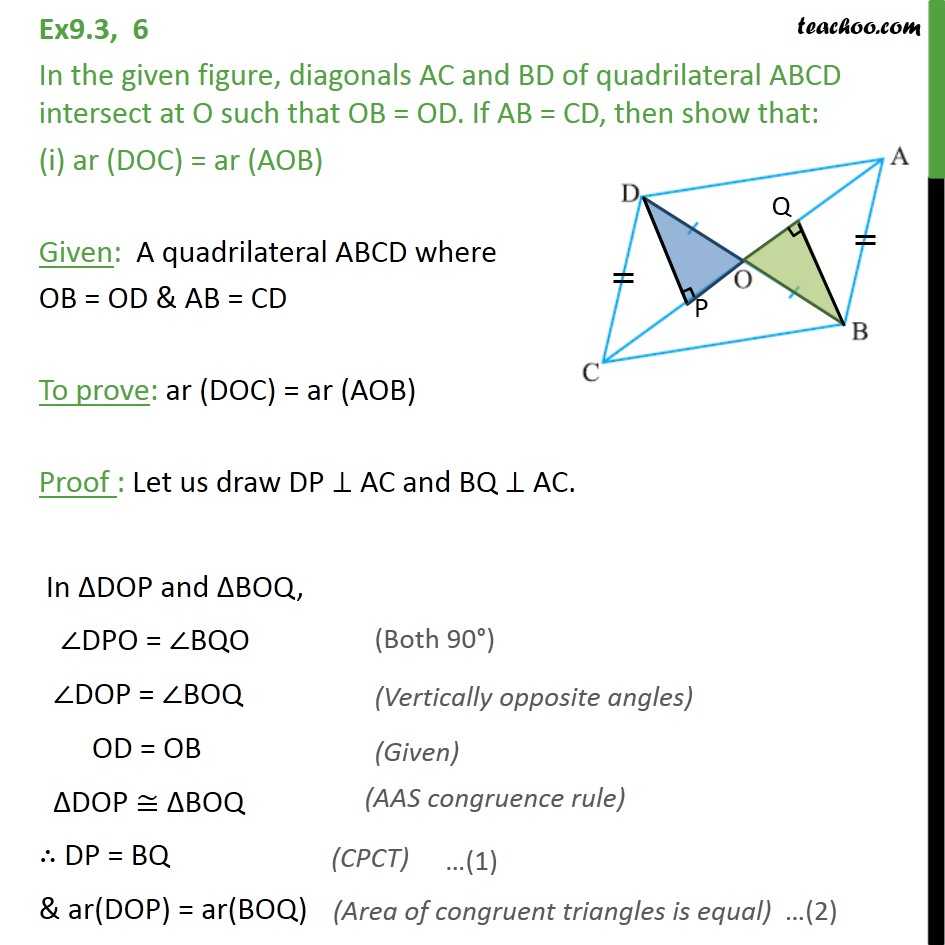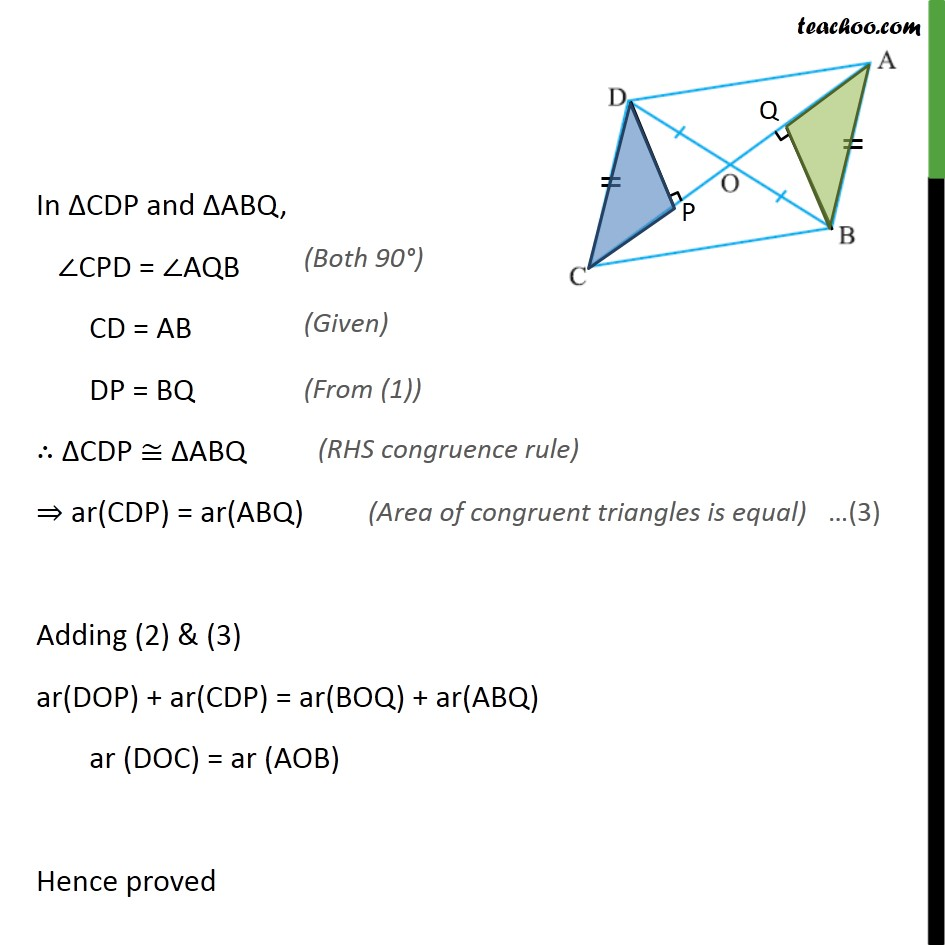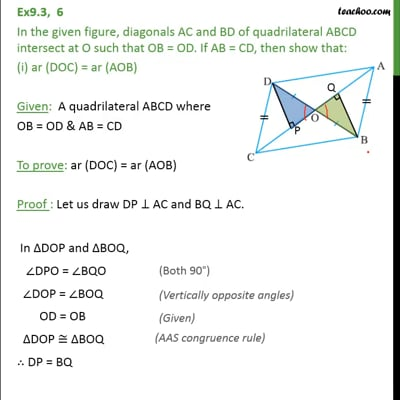This video is only available for Teachoo black users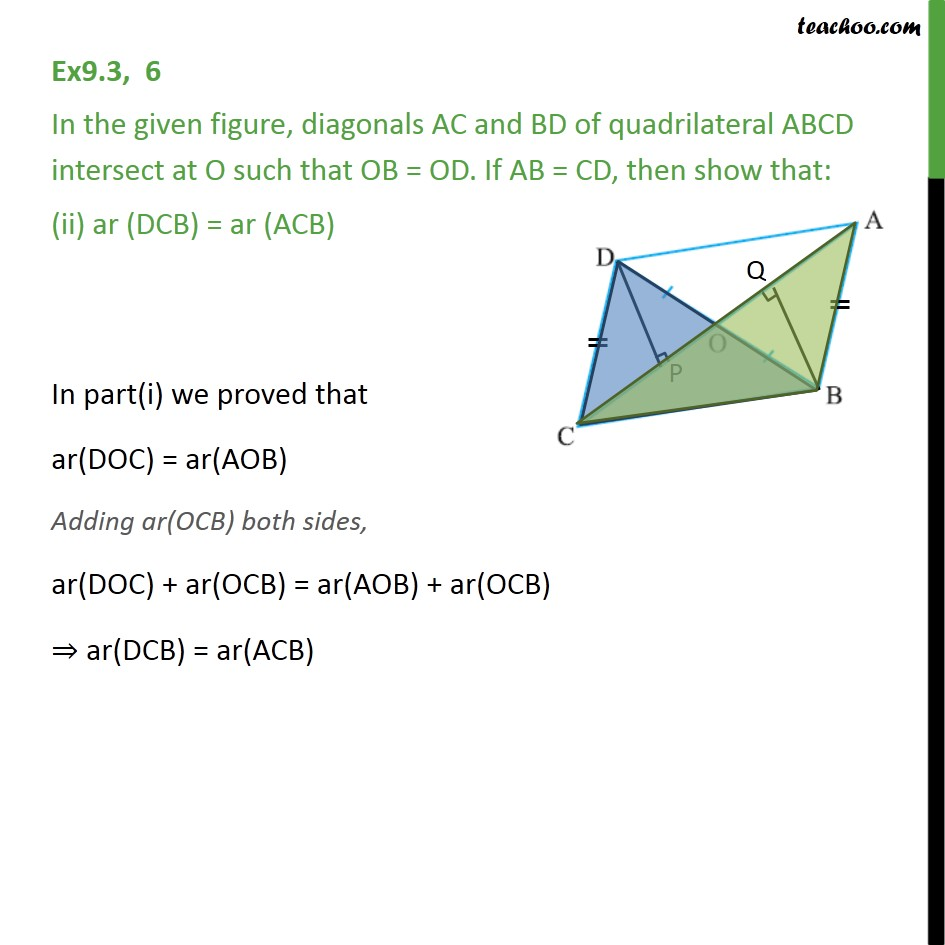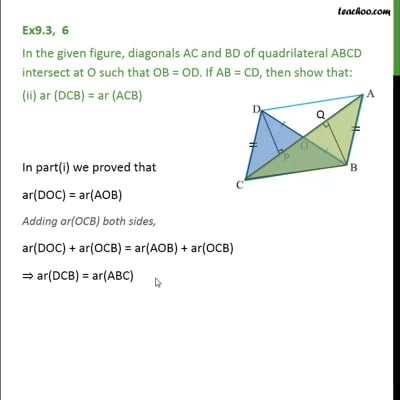This video is only available for Teachoo black users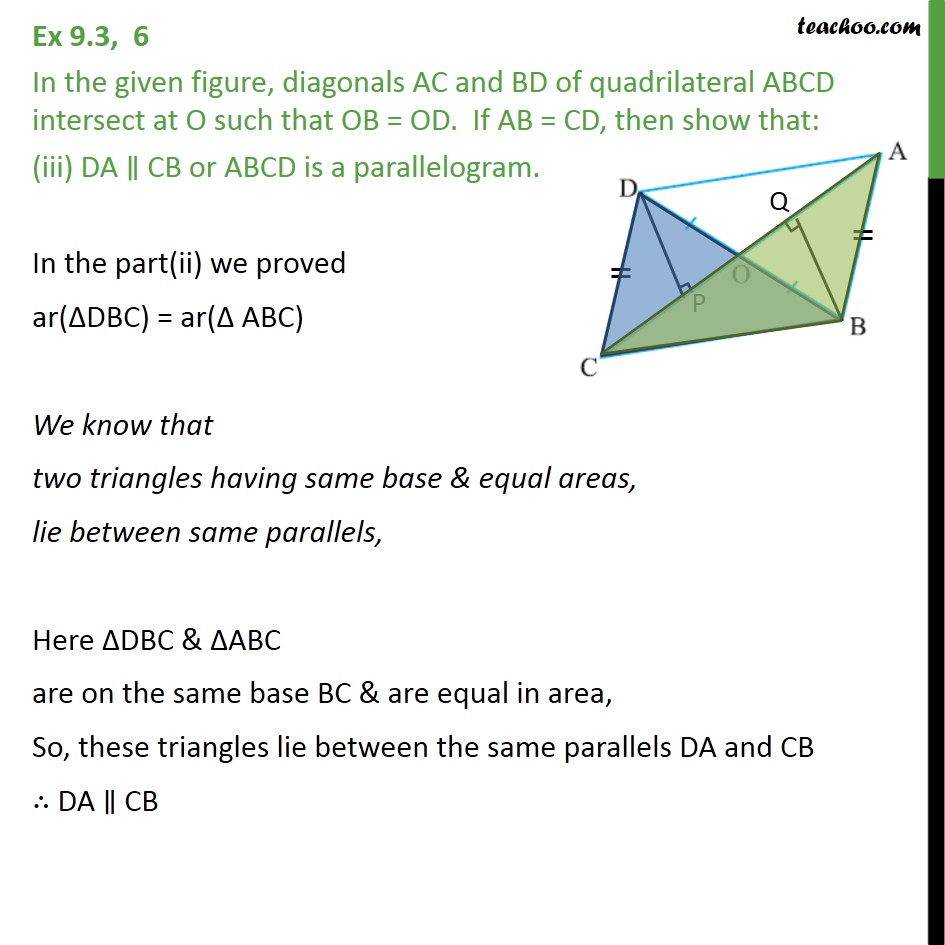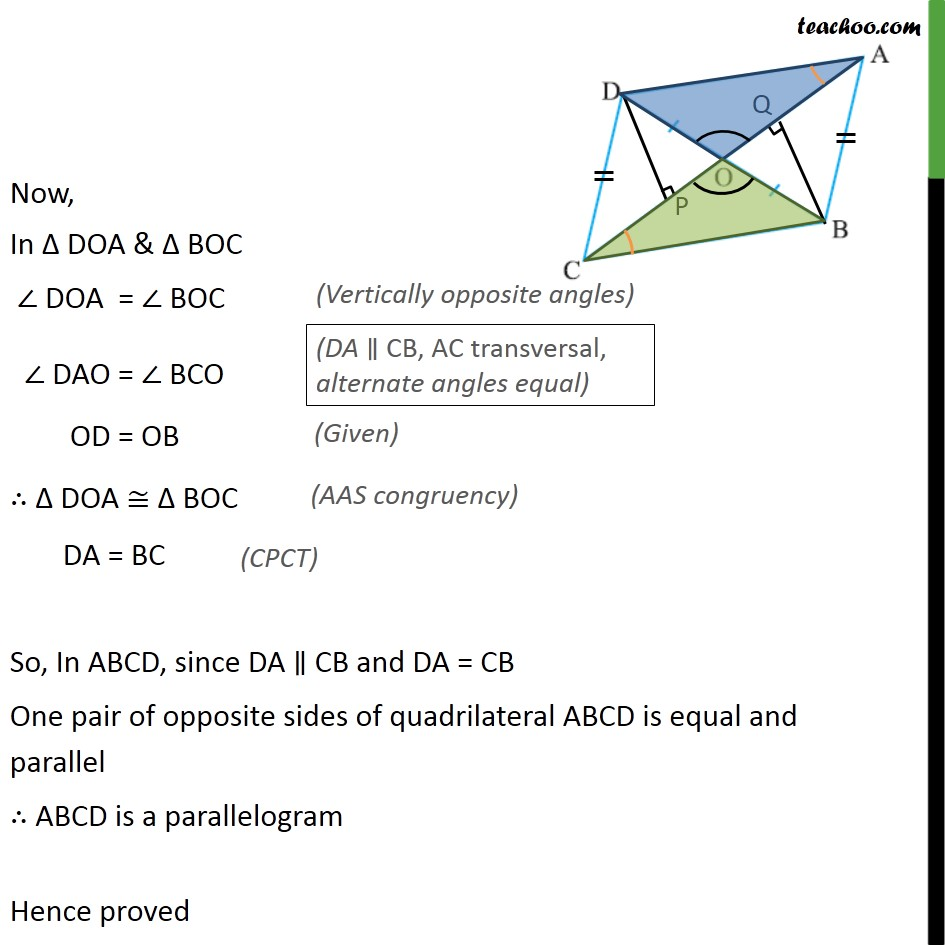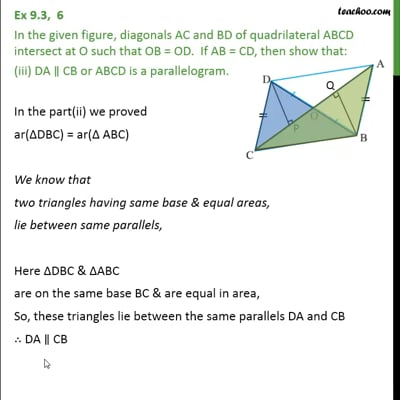This video is only available for Teachoo black users

### Transcript

Ex9.3, 6 In the given figure, diagonals AC and BD of quadrilateral ABCD intersect at O such that OB = OD. If AB = CD, then show that: (i) ar (DOC) = ar (AOB) Given: A quadrilateral ABCD where OB = OD & AB = CD To prove: ar (DOC) = ar (AOB) Proof : Let us draw DP AC and BQ AC. In DOP and BOQ, DPO = BQO DOP = BOQ OD = OB DOP BOQ DP = BQ & ar(DOP) = ar(BOQ) In CDP and ABQ, CPD = AQB CD = AB DP = BQ CDP ABQ ar(CDP) = ar(ABQ) Adding (2) & (3) ar(DOP) + ar(CDP) = ar(BOQ) + ar(ABQ) ar (DOC) = ar (AOB) Hence proved Ex9.3, 6 In the given figure, diagonals AC and BD of quadrilateral ABCD intersect at O such that OB = OD. If AB = CD, then show that: (ii) ar (DCB) = ar (ACB) In part(i) we proved that ar(DOC) = ar(AOB) Adding ar(OCB) both sides, ar(DOC) + ar(OCB) = ar(AOB) + ar(OCB) ar(DCB) = ar(ACB) Ex 9.3, 6 In the given figure, diagonals AC and BD of quadrilateral ABCD intersect at O such that OB = OD. If AB = CD, then show that: (iii) DA CB or ABCD is a parallelogram. In the part(ii) we proved ar( DBC) = ar( ABC) We know that two triangles having same base & equal areas, lie between same parallels, Here DBC & ABC are on the same base BC & are equal in area, So, these triangles lie between the same parallels DA and CB DA CB Now, In DOA & BOC DOA = BOC DAO = BCO OD = OB DOA BOC DA = BC So, In ABCD, since DA CB and DA = CB One pair of opposite sides of quadrilateral ABCD is equal and parallel ABCD is a parallelogram Hence proved